Evaluating machine learning algorithms, training set, cross validation set, test set, bias, variance, learning curves and improving algorithm performance.

## 1. Evaluating Learning Algorithm

I would like to give full credits to the respective authors as these are my personal python notebooks taken from deep learning courses from Andrew Ng, Data School and Udemy :) This is a simple python notebook hosted generously through Github Pages that is on my main personal notes repository on https://github.com/ritchieng/ritchieng.github.io. They are meant for my personal review but I have open-source my repository of personal notes as a lot of people found it useful.

### 1a. Deciding what to try next

• Suppose you have implemented regularized linear regression to predict housing prices
• However, when you test your hypothesis your hypothesis on new set of houses, you find that it makes unacceptably large errors
• You can do the following
• Get more training data
• Smaller set of features
• Try decreasing lambda
• Try increasing lambda
• Typically people randomly choose these avenues and then figure out it may not be suitable
• There is a simple technique to weed out avenues that are not suitable
• Machine Learning Diagnostic
• Test that you can run to gain insight what is or isn’t working with a learning algorithm and gain guidance as to how best to improve its performance
• Diagnostics can take time to implement, but doing so can be a very good use of your time
• But it’s worth the time compared to spending months on unsuitable avenues

### 1b. Evaluating a hypothesis

• In fitting parameters to your training data, you would want to lower your training error to the minimum
• How to tell if over-fitting?
• You can plot for few features
• For many features: training/testing procedure
• Split into 2 portions
• Training set
• Test set
• Randomly re-order data before splitting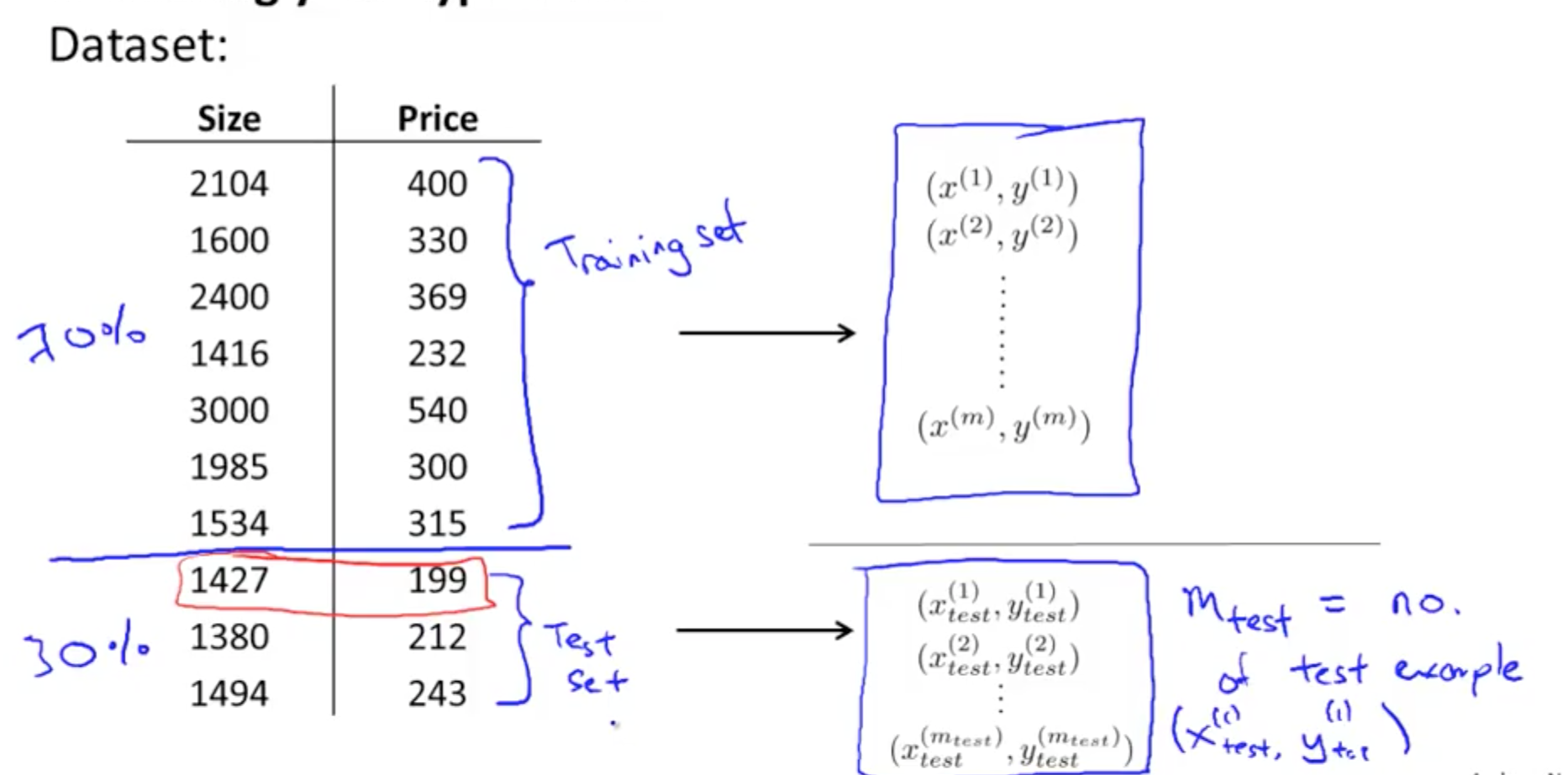• Training/testing procedure: linear regression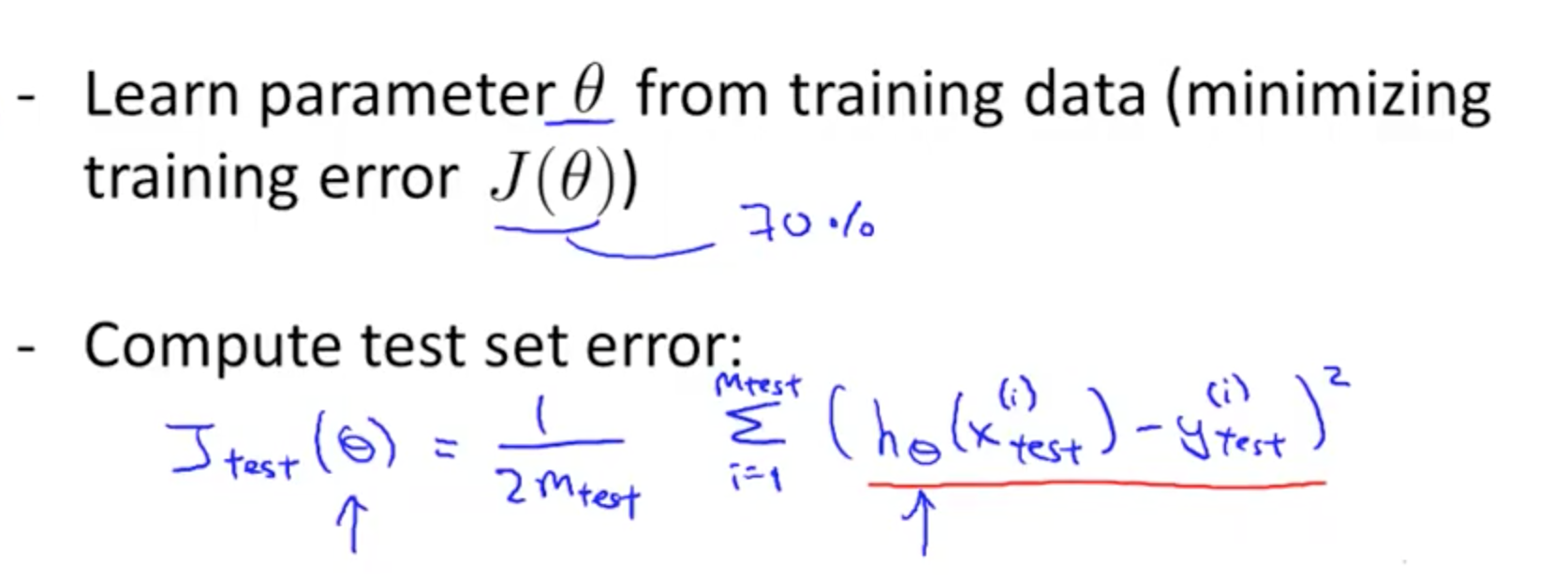• Training/testing procedure: logistic regression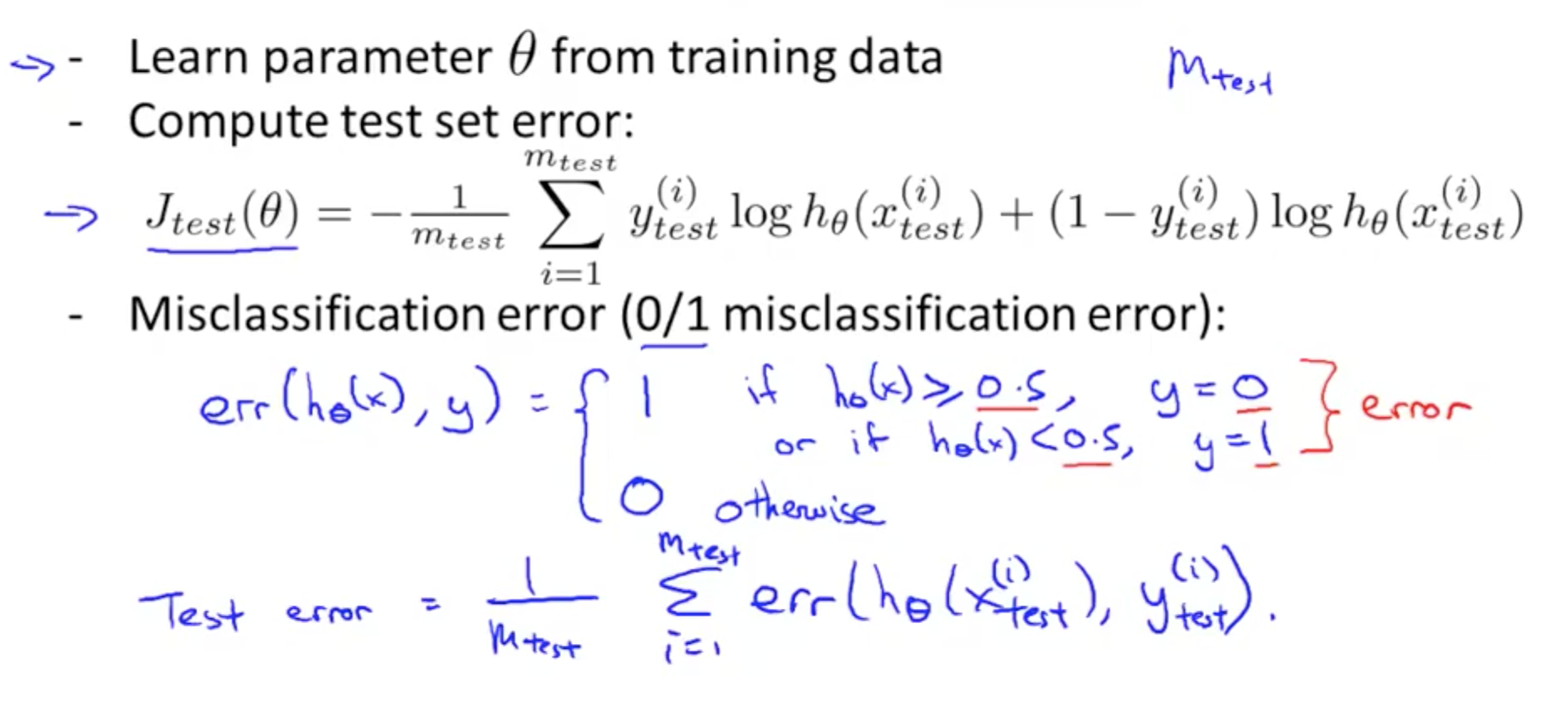### 1c. Model selection and Train/Validation/Test Sets

• Model selection
• We can create an extra parameter d which is the degree of polynomial
• You can measure the test error on each parameter θ
• If you choose d = 5 and to determine how well the model generalizes, you can report test set error on Jtest(θ5)
• But there is a problem: Jtest(θ5) is likely an optimistic estimate of generalization error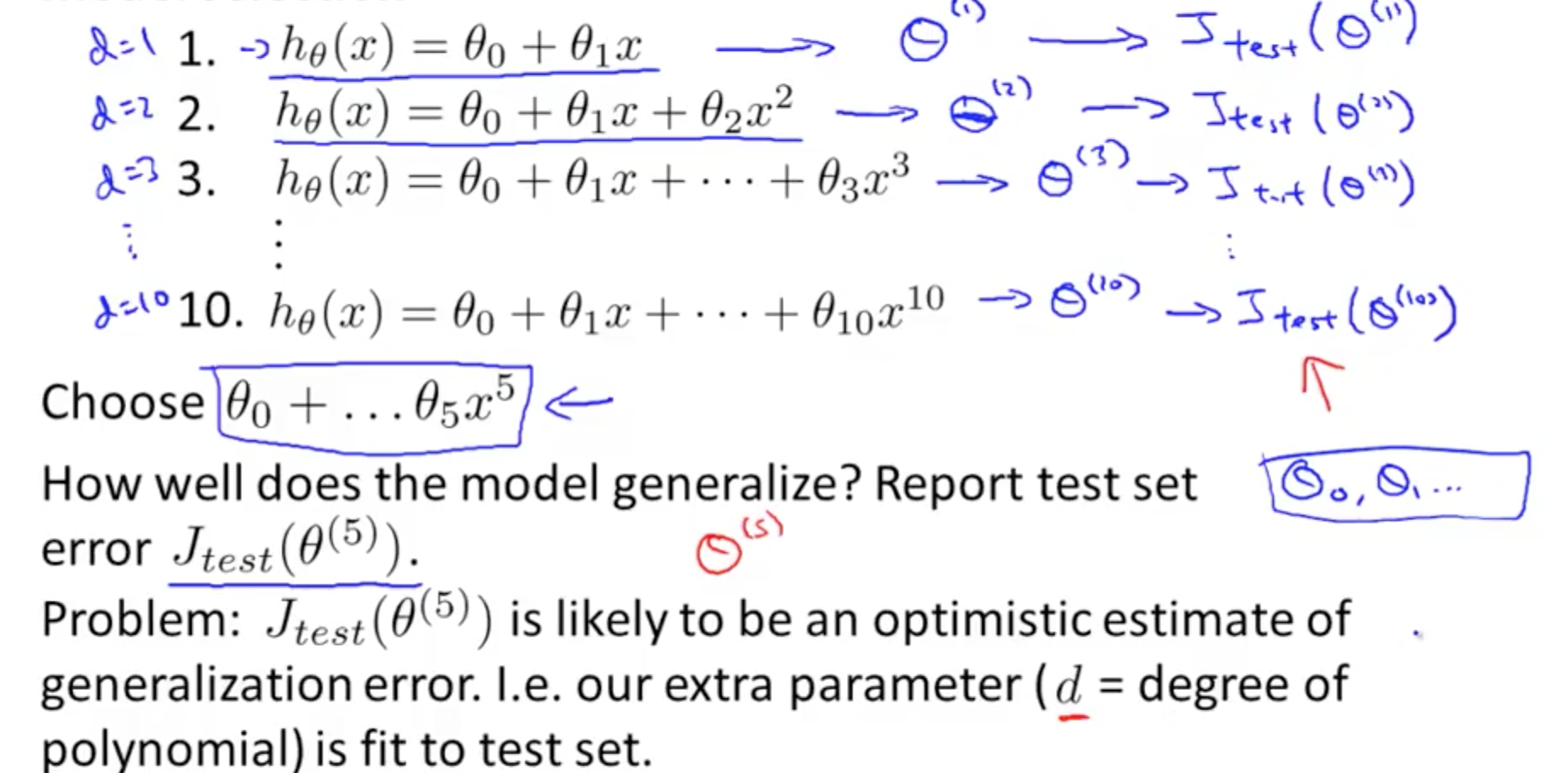• To address the problem, we can do the following
• Split data into 3 categories
• Training set
• Cross validation set or Validation set or CV
• Test set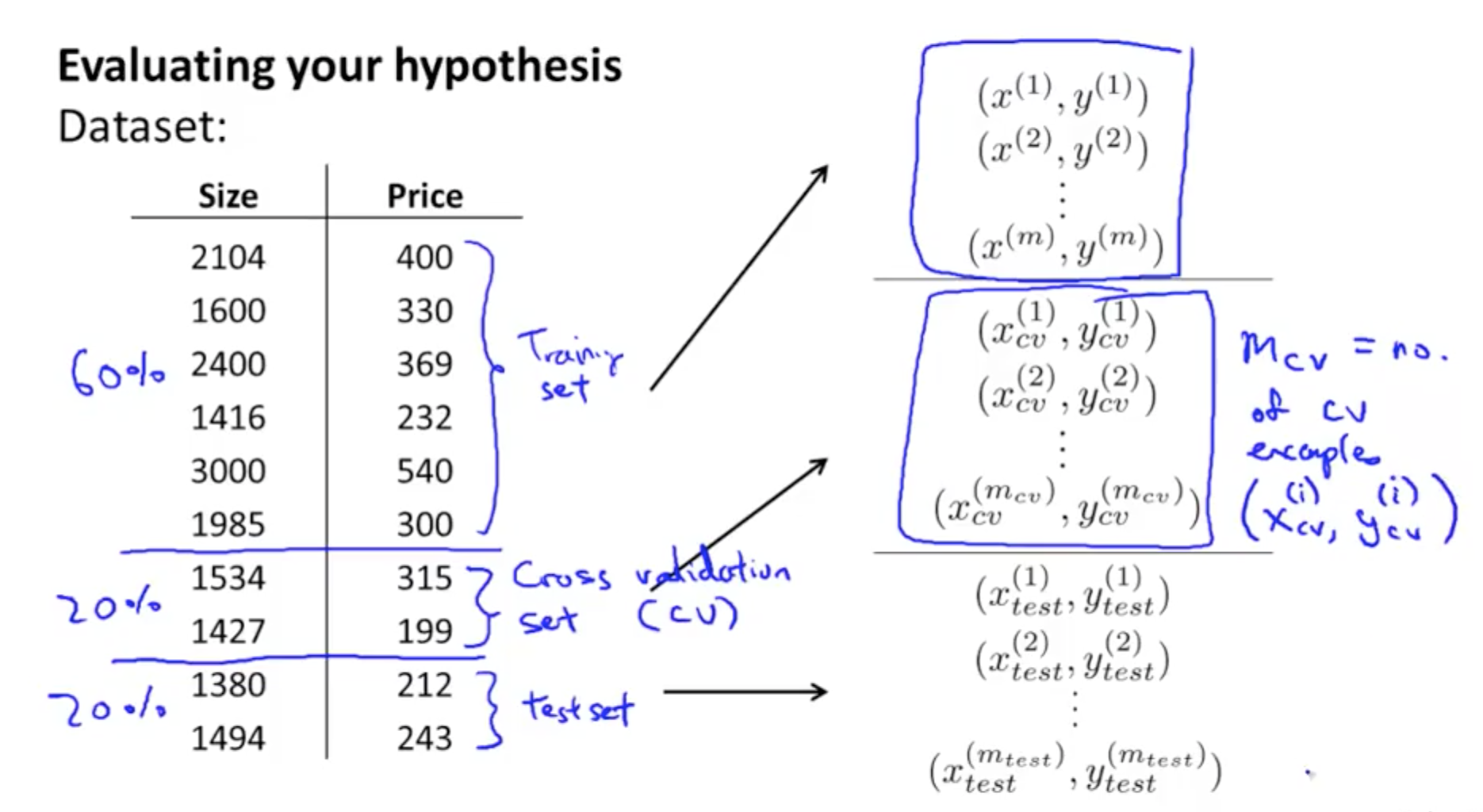• You would have the following 3 errors
• Training error
• Cross validation (CV) error
• Test error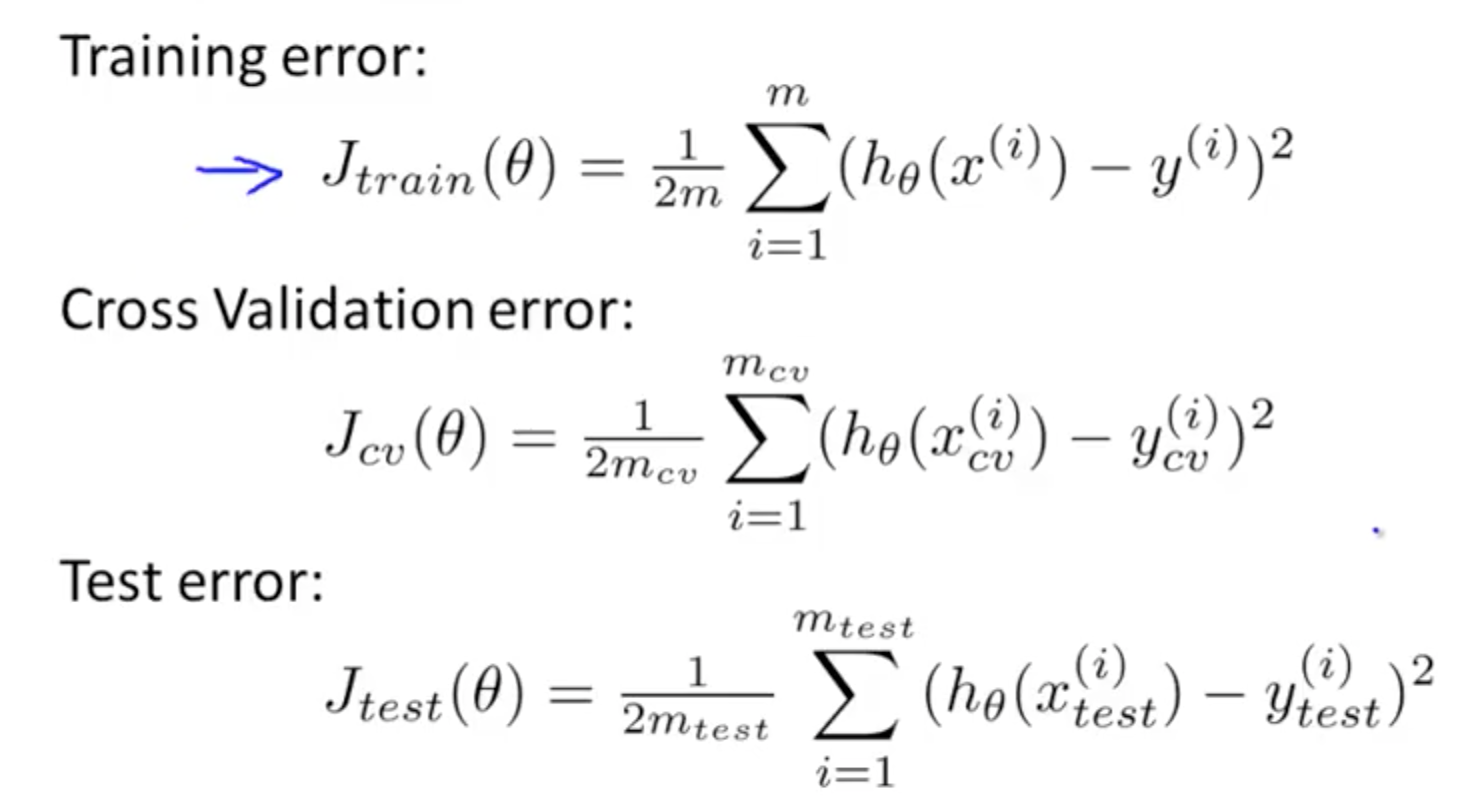• We would test on cross-validation sets
• Pick hypothesis with lowest CV error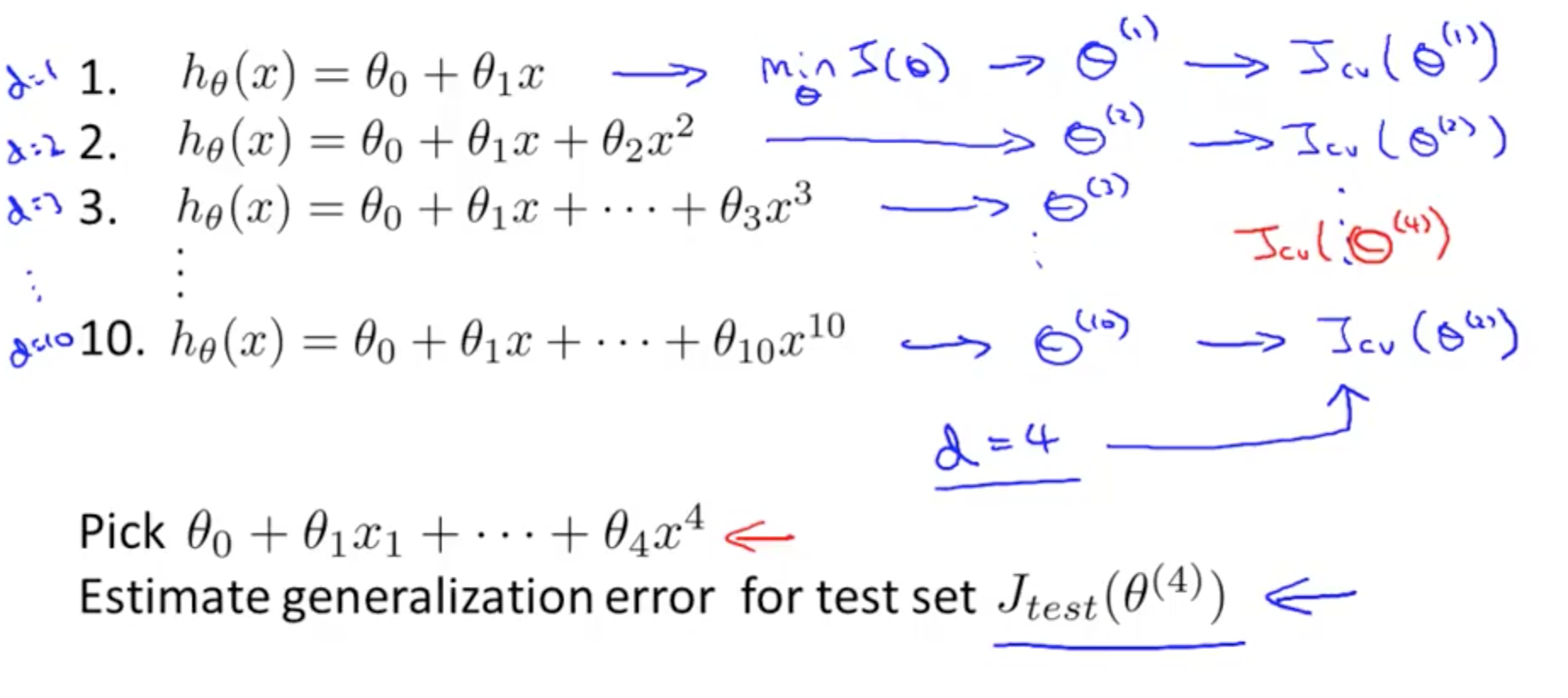## 2. Bias vs Variance

### 2a. Diagnosing vs Variance

• When you run an algorithm and it doesn’t do as well as you hope, it typically has a high bias or high variance issue
• High bias (underfitting)
• High variance (overfitting)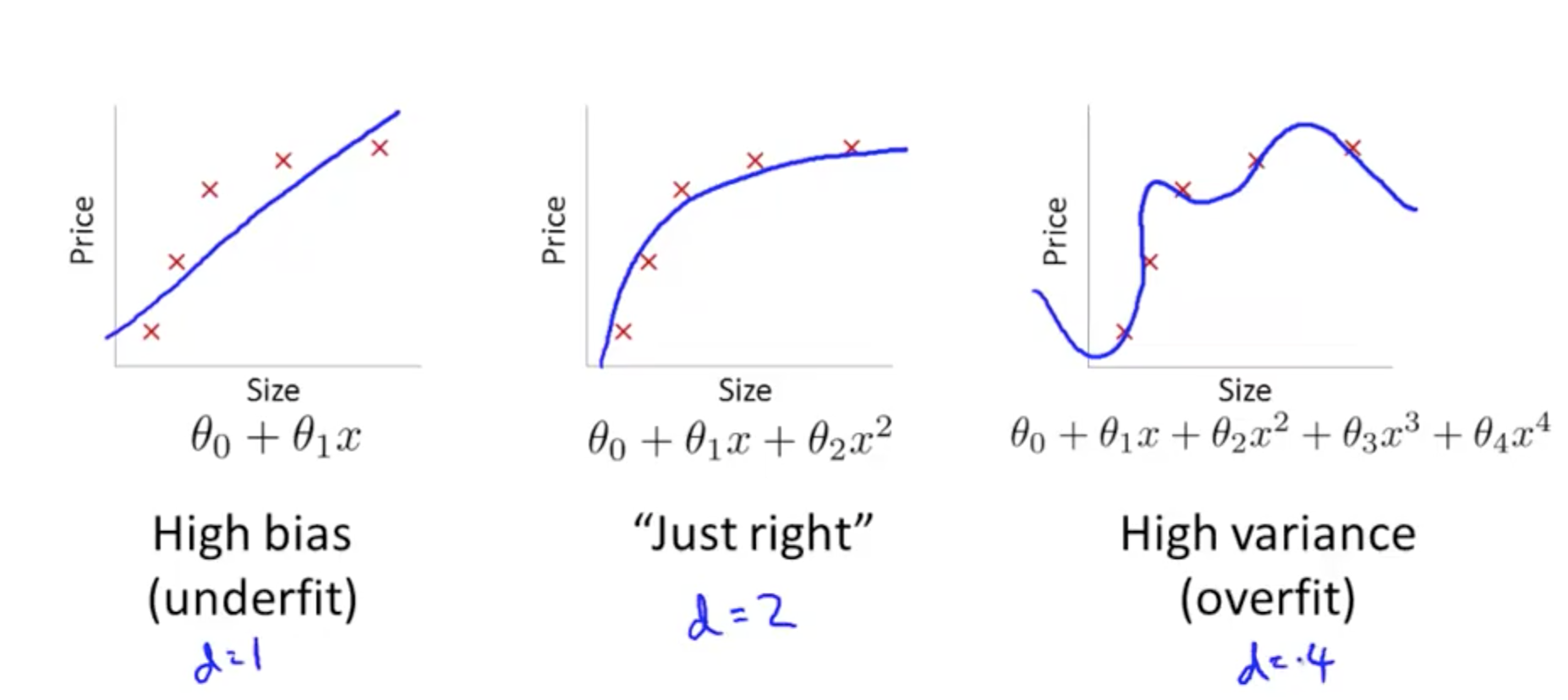• Plot error against degree of polynomial, d
• As you increase your polynomial, d,
• Training error decreases from underfitting to overfitting
• Cross validation (CV) error example
• d = 1: underfitting, high CV error
• d = 2: lower CV error due to better fit
• d = 4: overfitting, high CV error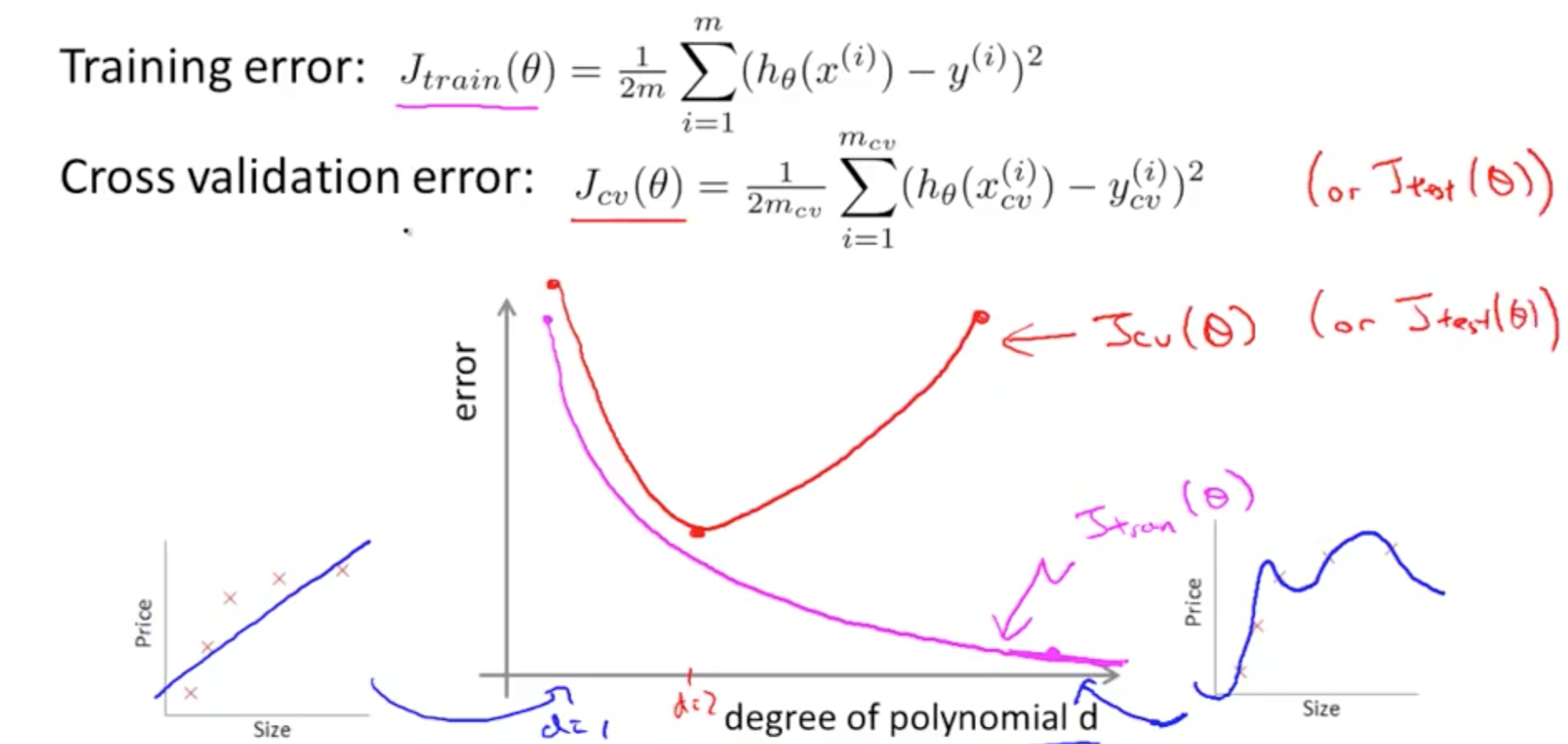• How do we distinguish between a high bias or a high variance issue?
• High Bias Error
• High Jtrain(θ)
• Jtrain(θ) = Jcv(θ)
• High Variance Error
• Low Jtrain(θ)
• Jcv(θ) » Jtrain(θ)
• Much greater as seen on the right of the graph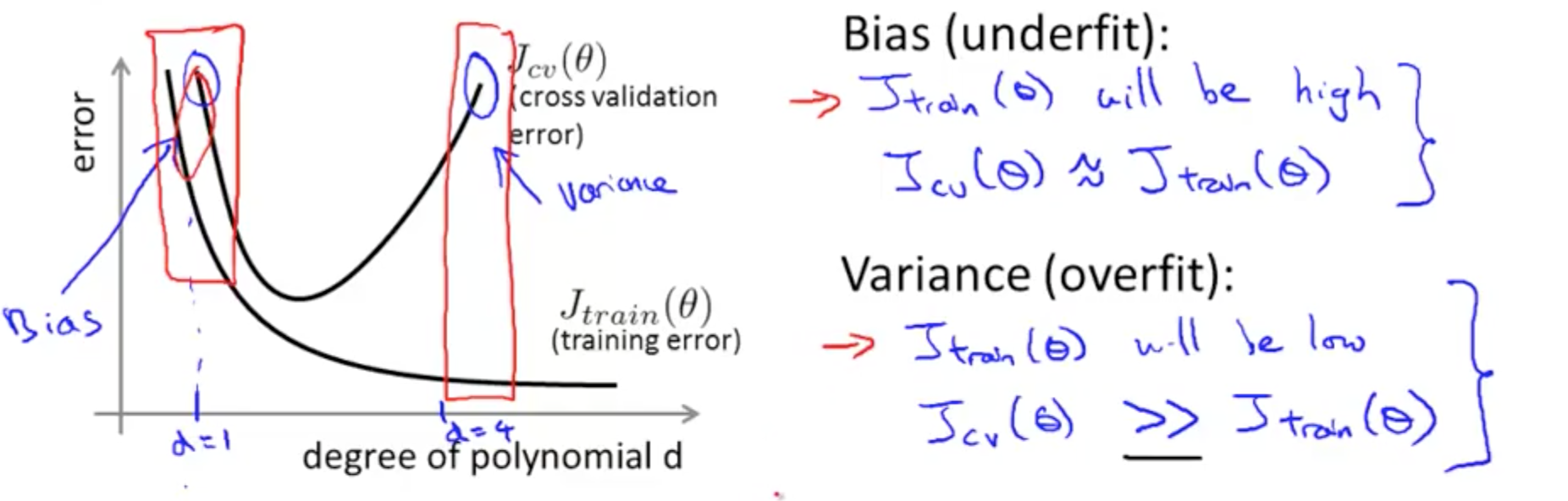### 2b. Regularization and Bias/Variance

• Linear regression with regularization
• Large λ
• High bias (underfit)
• Small λ
• High variance (overfit)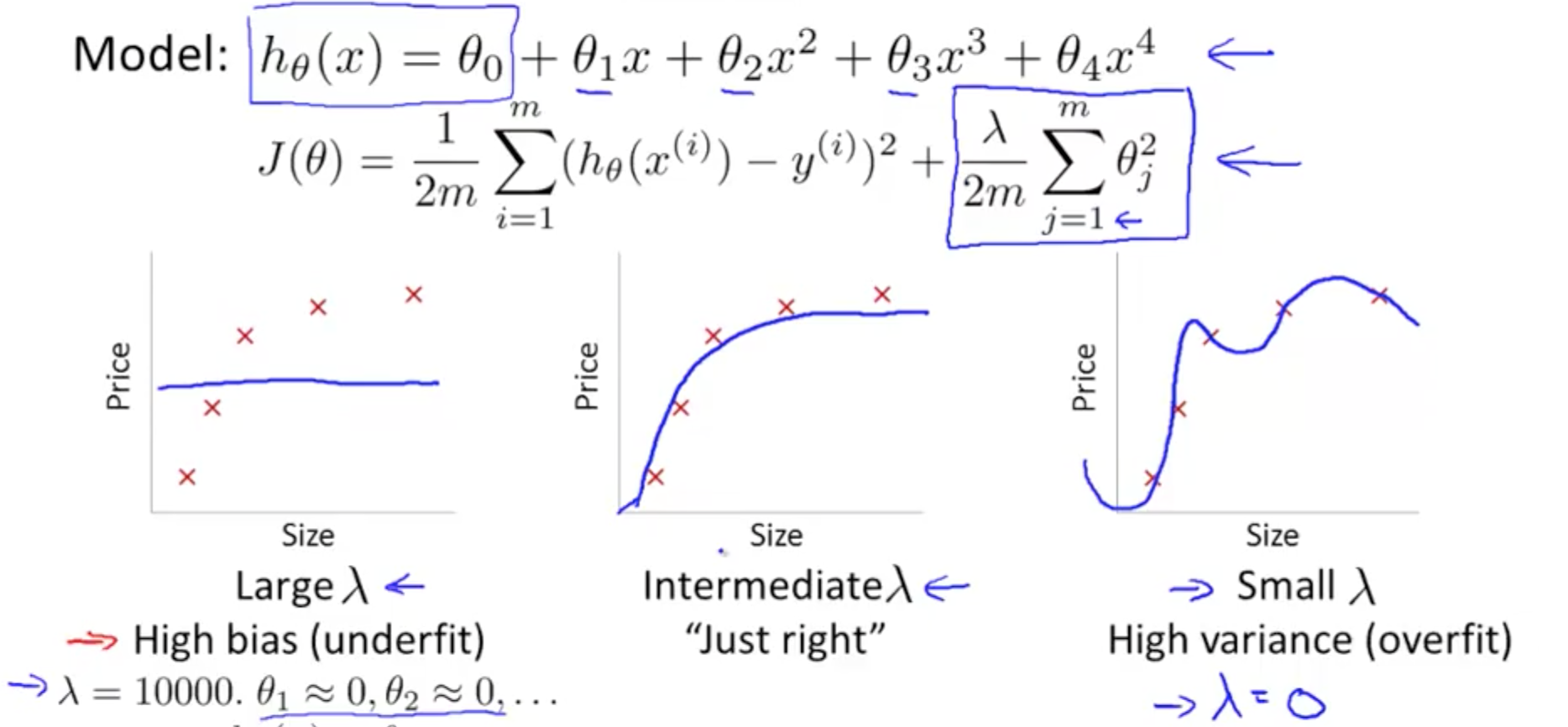• So how do we choose a good value of λ?
• H(θ): algorithm; hypothesis
• J(θ): cost function; optimization objective
• Jtrain(θ), Jcv(θ), Jtest(θ): optimization objectives without regularization terms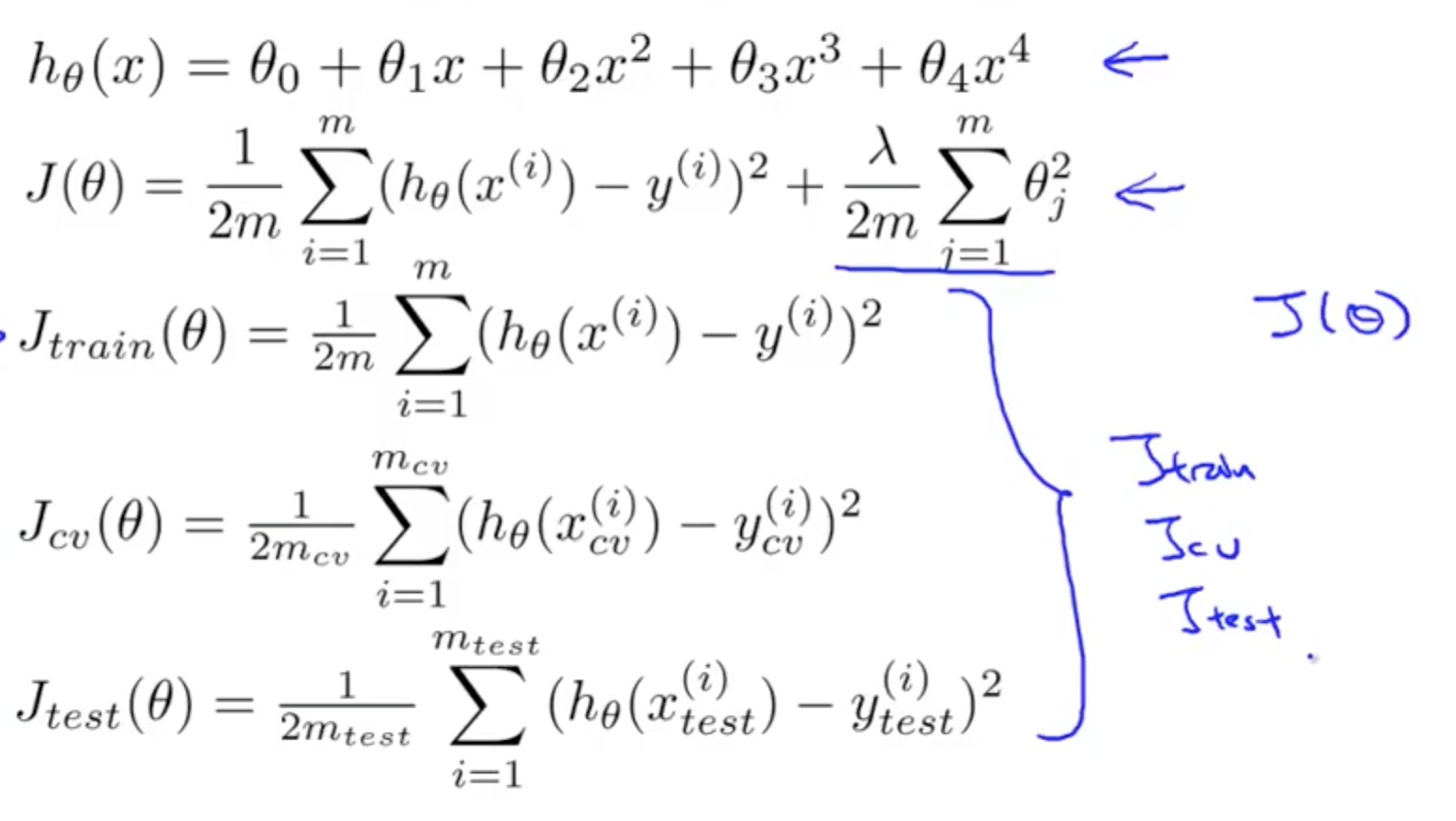• Steps
• Try λ in multiples of 2 on J(θ)
• Minimise J(θ) to get θ
• Try λ in multiples of 2 on Jcv(θ)
• Minimise Jcv(θ) to get θ
• Choose lowest Jcv(θ), θ_low
• Where θ_low is θ_5 in the example since Jcv(θ_5) is the lowest
• Pick Jcv(θ), θ_low
• Try for Jtest(θ_low)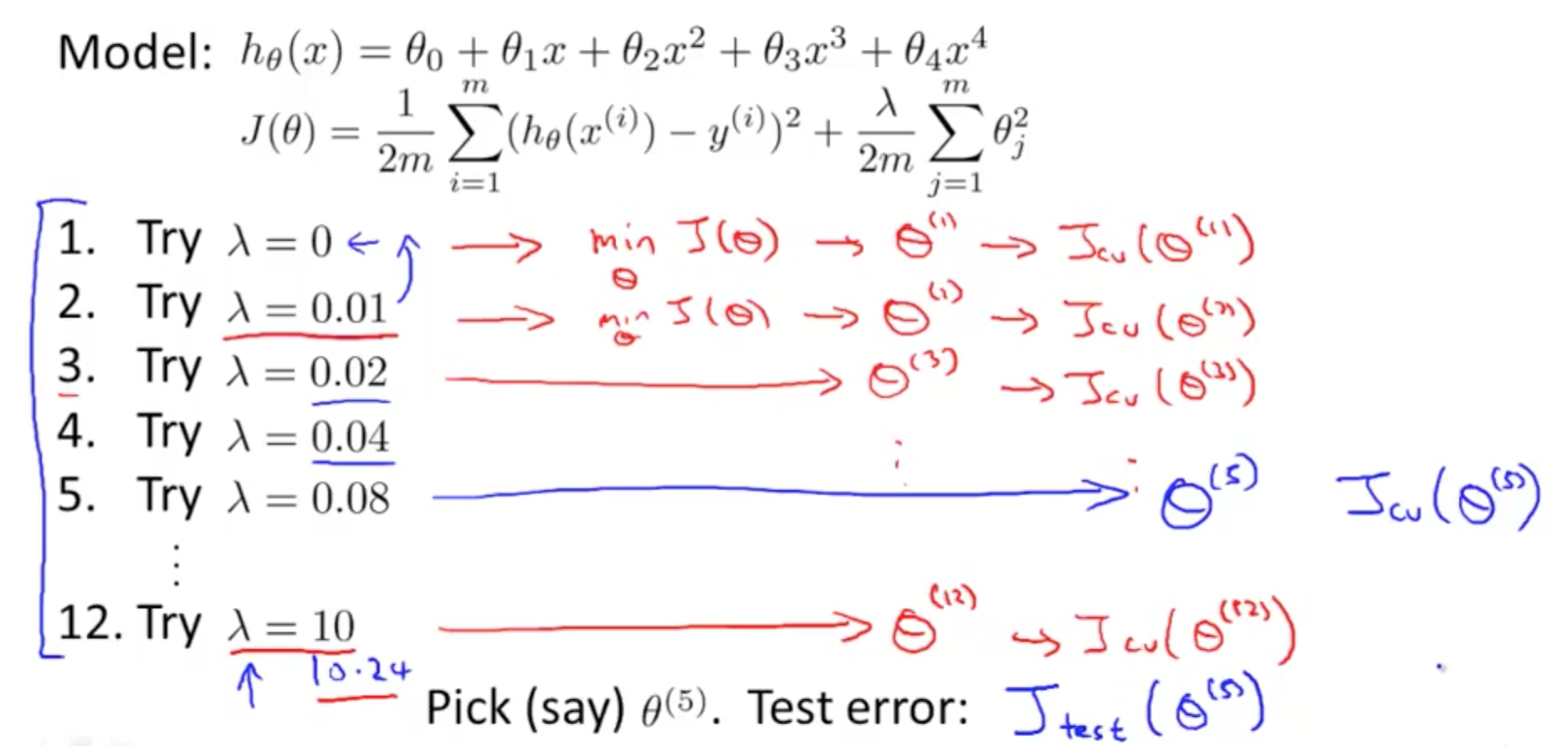• How CV and test error vary as we vary λ?
• Jtrain(θ)
• Small λ
• Regularization term is small
• Hypothesis fits better to the data
• Low Jtrain(θ)
• Large λ
• Regularization term is large
• Hypothesis does not fit well to the data
• High Jtrain(θ)
• Jcv(θ)
• Large λ
• Regularization term is large
• High bias (underfitting)
• Large Jcv(θ)
• Small λ
• Regularization term is small
• High variance (overfitting)
• Small Jcv(θ)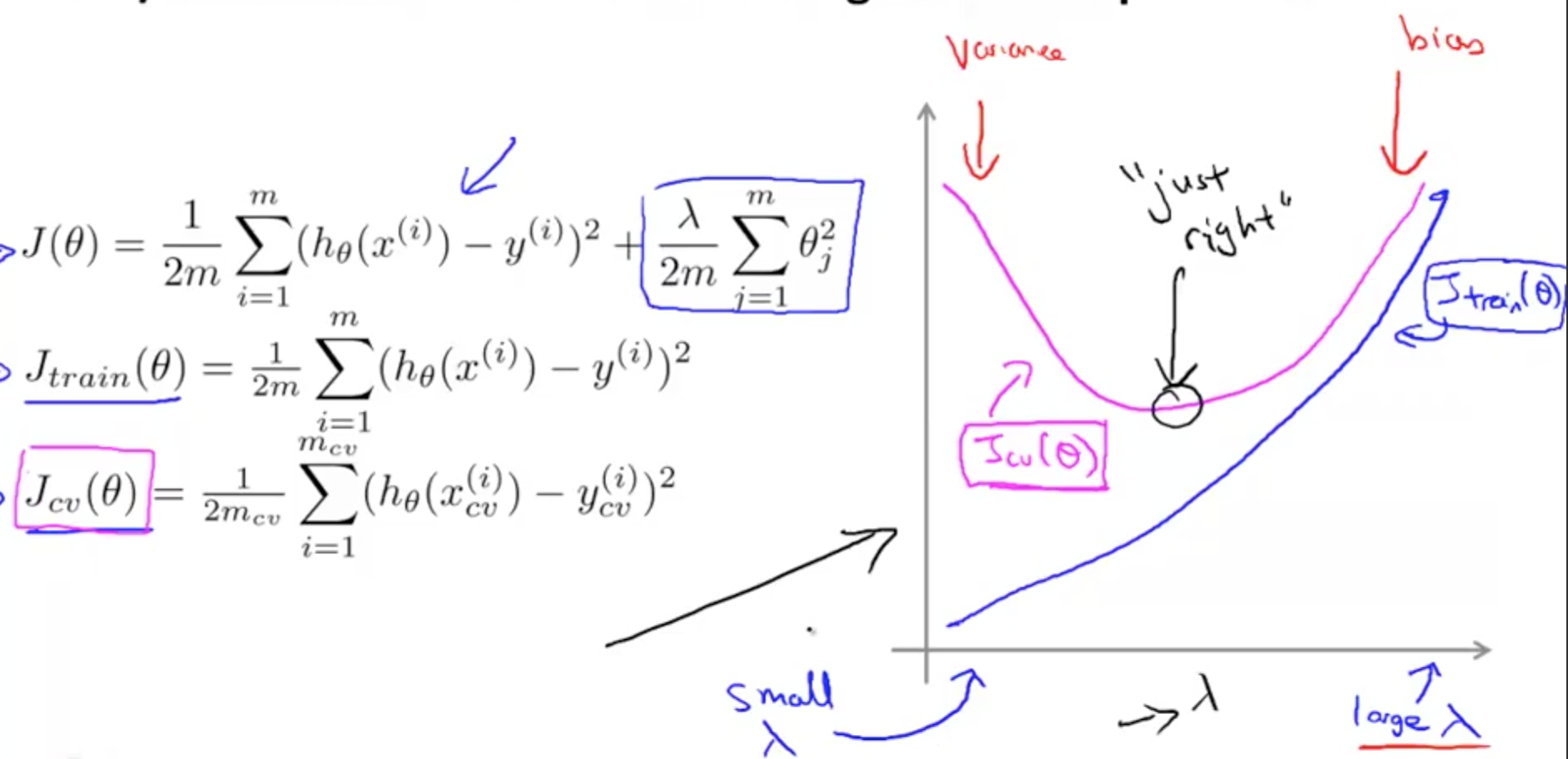• For a real dataset, the graph is messier, but the general trend is similar

### 2c. Learning Curves

• What is the effect of m, number of training examples, on training error?
• For m = 1, 2, 3 in the example
• If the training set is small
• Easier to fit every single training example perfectly
• Your training error = 0 or small
• For m = 4, 5, 6
• If the training set grows larger
• Harder to fit every single training example perfectly
• In general, when m increases, training error increases
• What is the effect of m, number of training examples, on cross validation error?
• The more data you have, where m increases
• Your cross validation error decreases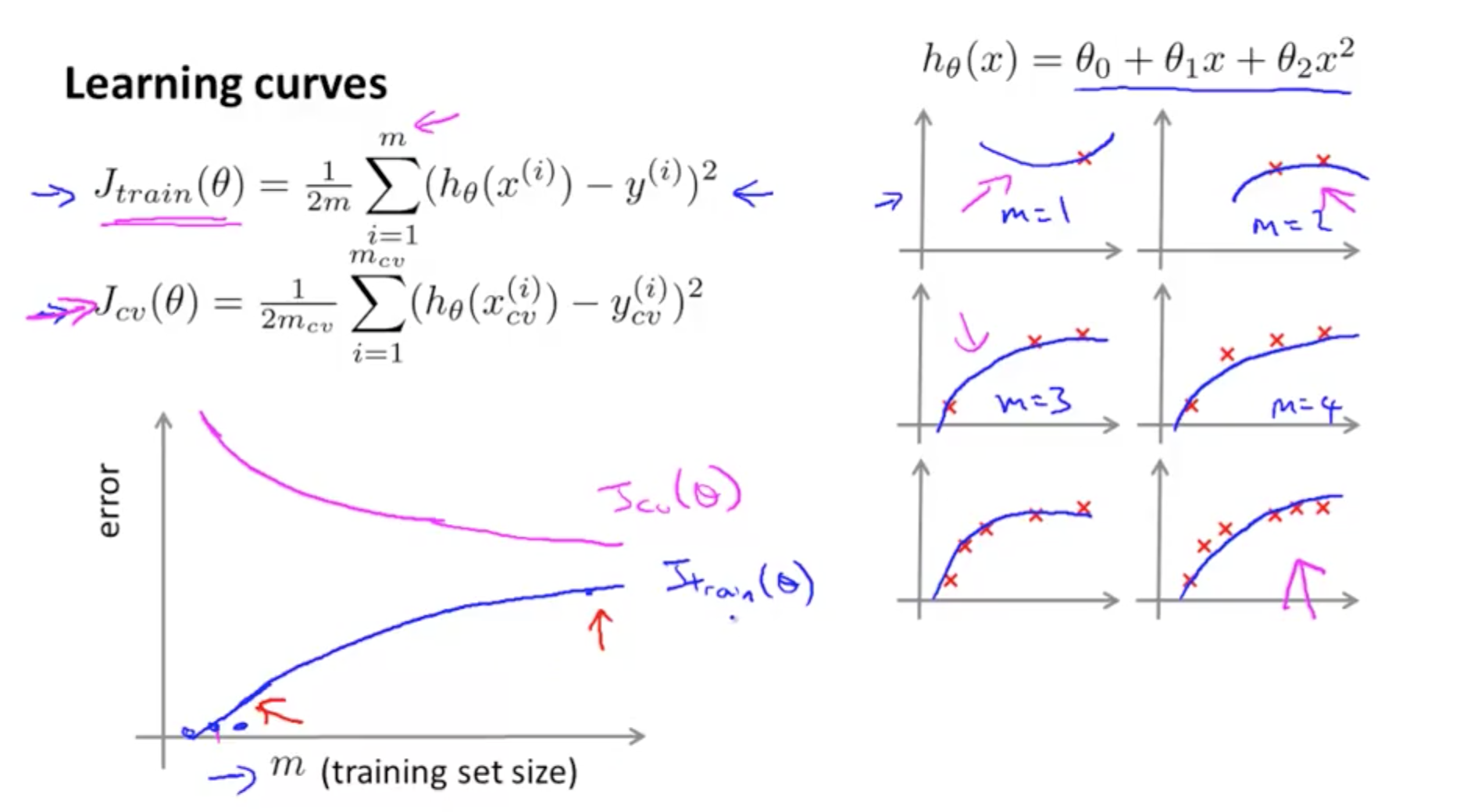• High Bias (Underfit)
• Poor performance on both training and test sets
• Your cross validation error decreases, but it decreases to a high value
• Even if you have large m, you still have a straight line with a high bias
• Your cross validation error would still be high
• Your training error increases close to the level achieve from your cross validation error
• If a learning algorithm is suffering from high bias, getting more training data will not (by itself) help much
• As seen from the two graphs, even with a higher m, there’s no use collecting more data to decrease your cross validation error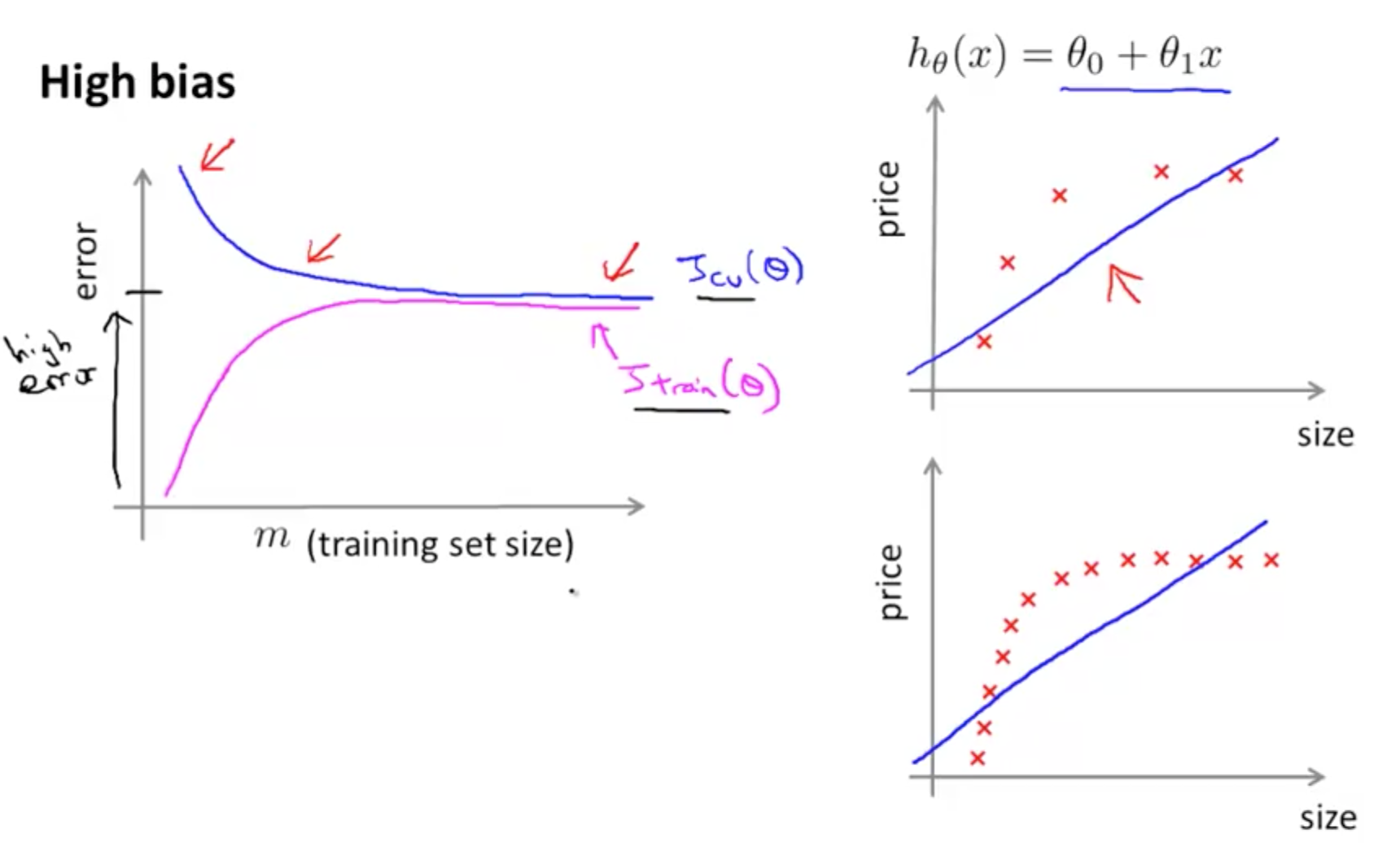• High Variance (Overfit)
• Gap in errors where training error is low but test error is high
• Training error would remain small
• This happens when you use a small λ
• Your training error increases with m because it becomes harder to fit your data
• Cross validation error would remain high
• This happens when you use a small λ
• If a learning algorithm is suffering from high variance, getting more data is likely to help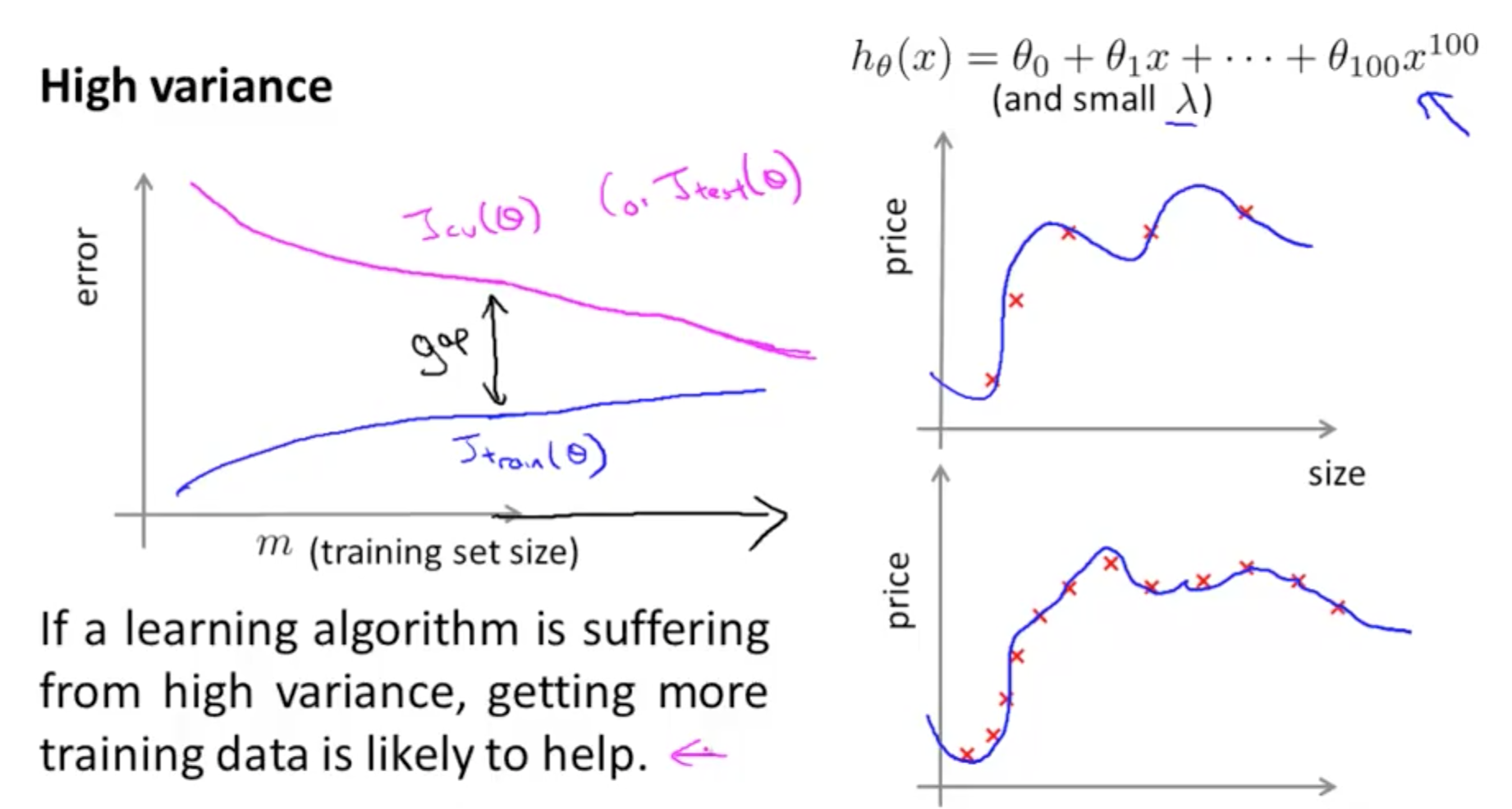### 2d. Improving Algorithm Performance

• Suppose you have implemented regularized linear regression to predict housing prices
• However, when you test your hypothesis your hypothesis on new set of houses, you find that it makes unacceptably large errors
• You can do the following
• Get more training data
• Fixes high variance
• Smaller set of features
• Fixes high variance
• Features are too complicated
• Fixes high bias
• Features are too simple
• Fixes high bias
• Too low d
• Try decreasing lambda
• Fixes high bias
• Because you would have a smaller regularized term, giving more importance to other features
• Try increasing lambda
• Fixes high variance
• Because you would have a larger regularized term, giving less importance to other features
• Neural Networks and Overfitting
• If you are fitting a neural network, you can use a small or large neural network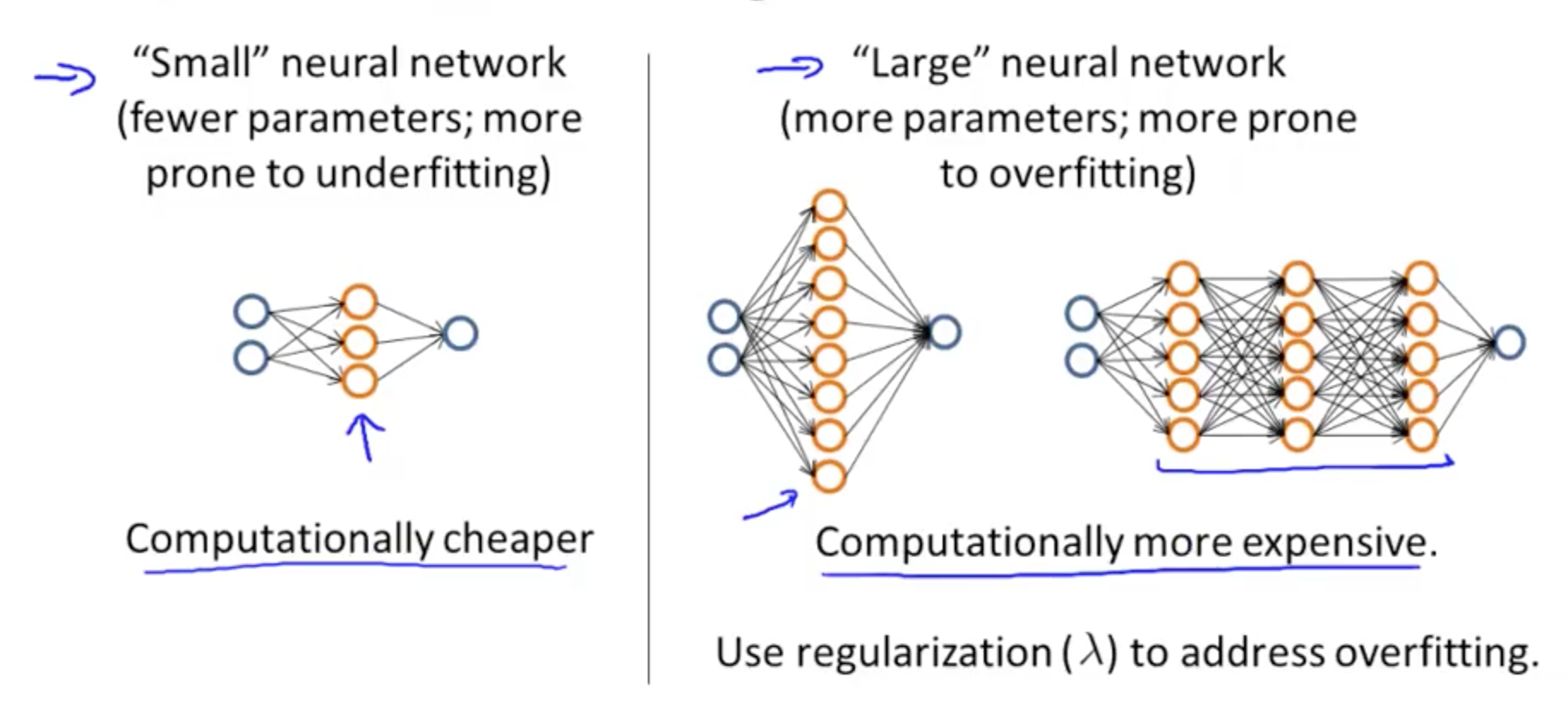• Small neural network
• 1 hidden layer
• 1 input layer
• 1 output layer
• Computationally cheaper
• Large neural network
• Multiple hidden layers
• 1 input layer
• 1 output layer
• Computationally expensive
Tags: